Solved

# Errors with operators

• 12 replies
• 385 views

• Newcomer
• 9 replies
I tried to multiply a set to a variable in my constraint, which gave the error There is no operation defined for operator "*" with the arguments of data type numeric and data type set. I need to find a solution to avoid this multiplication but come to the same result.

sum[j,Stationen*Zuordnung_AufgabenStationen(i,s)]-sum[i,Stationen*Zuordnung_AufgabenStationen(j,s)]<=0
icon

Best answer by mohansx 17 September 2019, 23:11

View original

This topic has been closed for comments

### 12 replies

Userlevel 5+5
Hello @Melike , what do you intend to do by the constraint you wrote ? If you can tell me that, I can tell you the right way to model that constraint.
Hello MOhan, Im trying to write a model for Assembly Line Balancing. The aim is to minimize the number of stations on the assembly line. In that constraint I was trying to model the precedence relations between the tasks. Zurodnung_AufgabenStationen is the assignment of tasks to stations. j is the predecessor task.
Userlevel 5+5
@Melike , do you mean - for all Stationen, the precedence relations must be satisfied ? s is an index in the Stationen set, correct ?

In the case, remove the Stationen* inside the sum and put s as the index domain of the constraint. Something like the screenshot below. This will be interpreted as "for all stations s, sum[j, Zourdnun...(j,s)] - sum[i, Zoutdnu...(i,s)] must be less than or equal to 0. (Ignore the colors of the text, see the highlighted s in the Index domain.. field)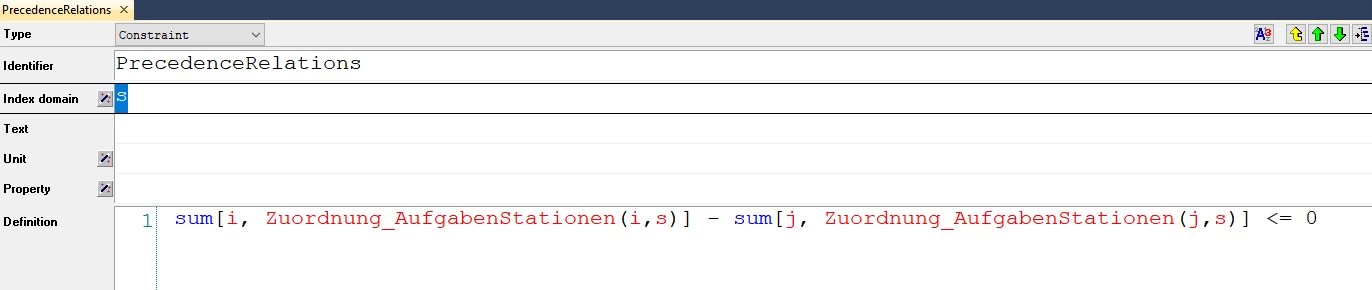Hello Mohan. "do you mean - for all Stationen, the precedence relations must be satisfied ? s is an index in the Stationen set, correct ?" Exactly. Thanks a lot no errors now.

I try to model the Stationnumber (Variable which is to minimize). I found a function Max(..) but didnt understand how to use it without giving the elements.
and then try to model it in another way
sum[(i,s),Stationen*Zuordnung_AufgabenStationen(I,s)]; I is the last Task. but I dont know how to enter the last Task. I would probably have to use the Max function again which would be redundant, because i would then use it for Stationnumber. And then again the problem with the multiplication would occur.
Userlevel 5+5
@Melike , can you elaborate what does Stationnumber represents ?

Do you want to minimize the total number of stations used ?

Again, sum[(i,s),Stationen*Zuordnung_AufgabenStationen(I,s)] is incorrect. Stationen is a set, and you cannot multiply a set in a constraint or a variable.

If you want to sum Zuordnung_AufgabenStationen for only the last Task l, then something like below could work.

My answer might not be what you are looking for because I don't have a proper understanding of your problem statement and how you have set up the different sets and variables, so sharing that would be helpful if you need more assistance.
I used Last(SetStations) and its working as i have expected. Thanks a lot.
I havent add the data yet and just now tried to add the data to the Tasks,but it didnt except it with the error "There is no operation defined for the operator = with arguments of data type numeric and data type element,arguments Number of Stations and last(Stations)". Aufgaben=Tasks .Stationsanzahl=Number of Stations. Zuordnung_AufgabenStationen=Assignment of tasks to Stations. Aufgabenzuordung= constraint for the task assignment. I send the picture for the variable Number of Stations and Tasks. j is the predecessortask.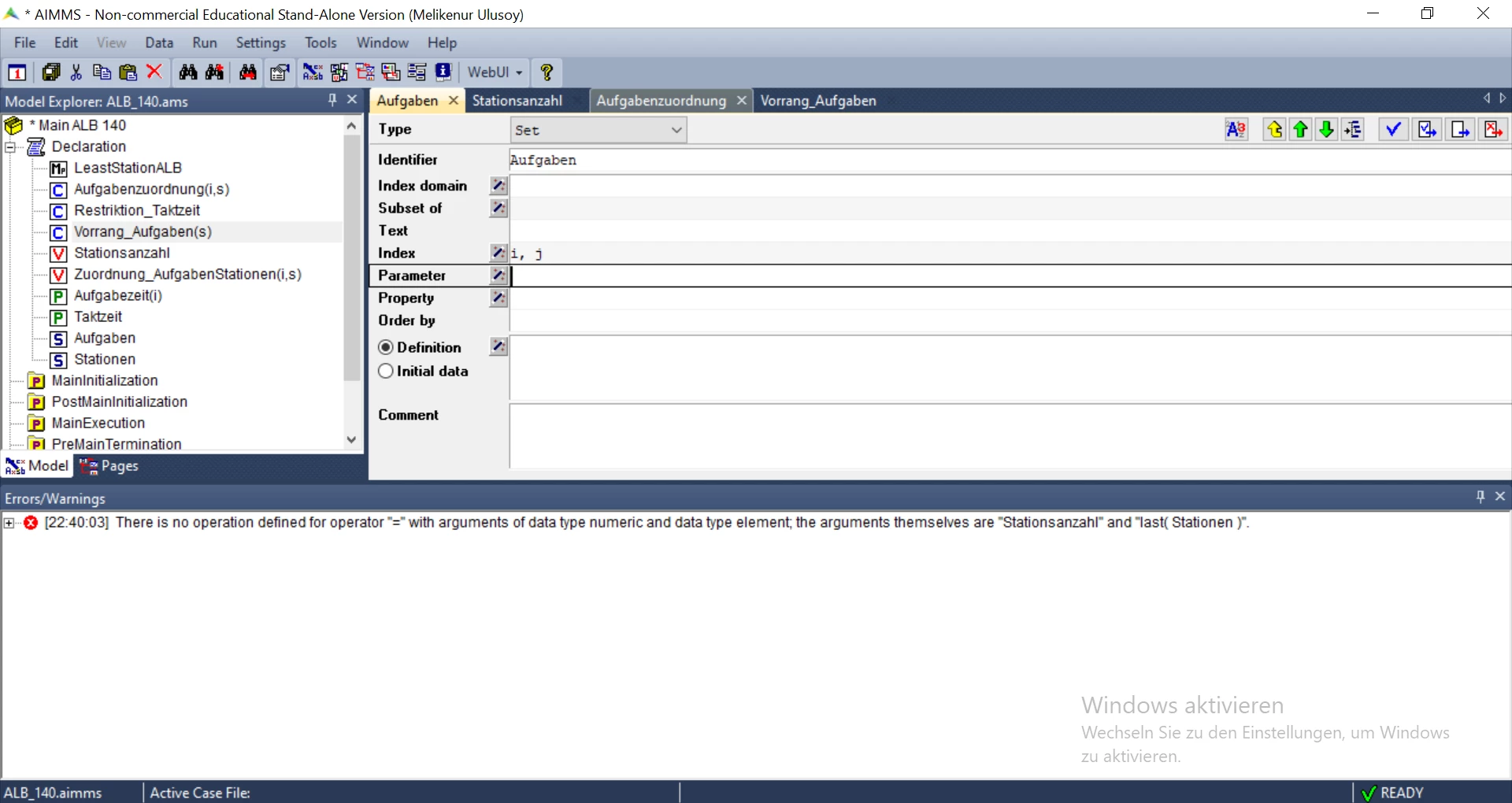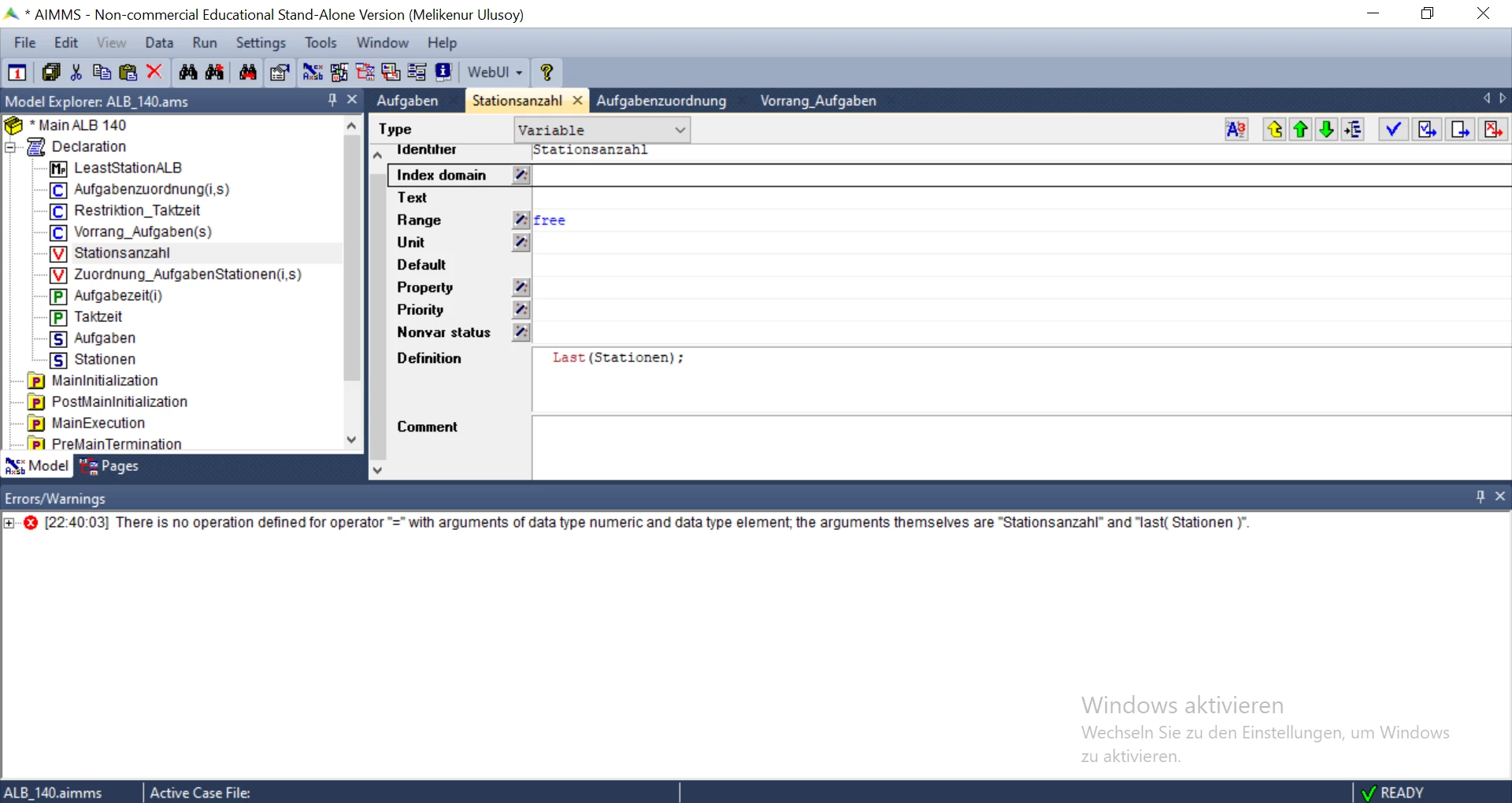Restriktion Taktzeit=Constraint for Cycletime
I m trying to minimize the number of stations(Stationsanzahl)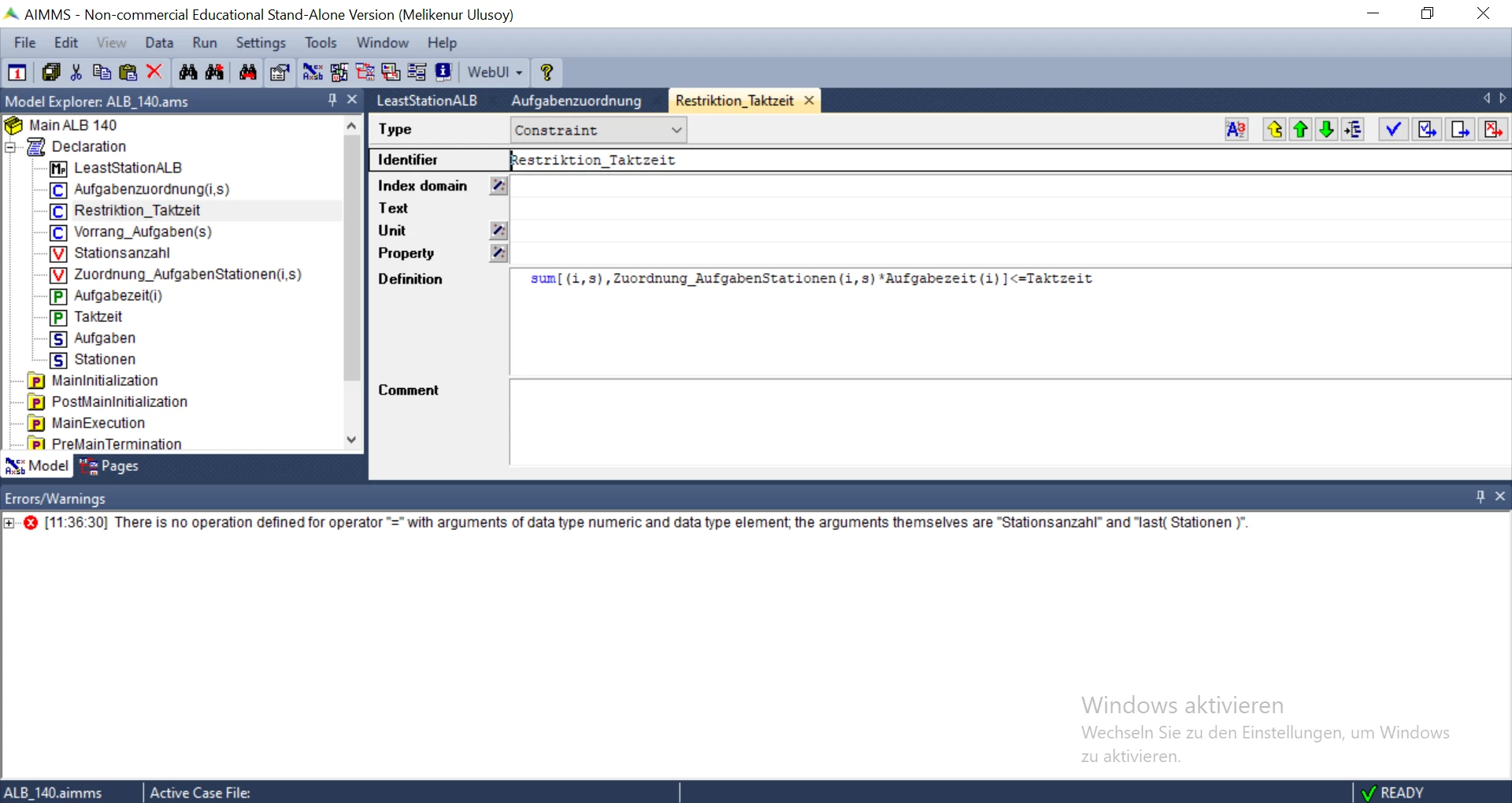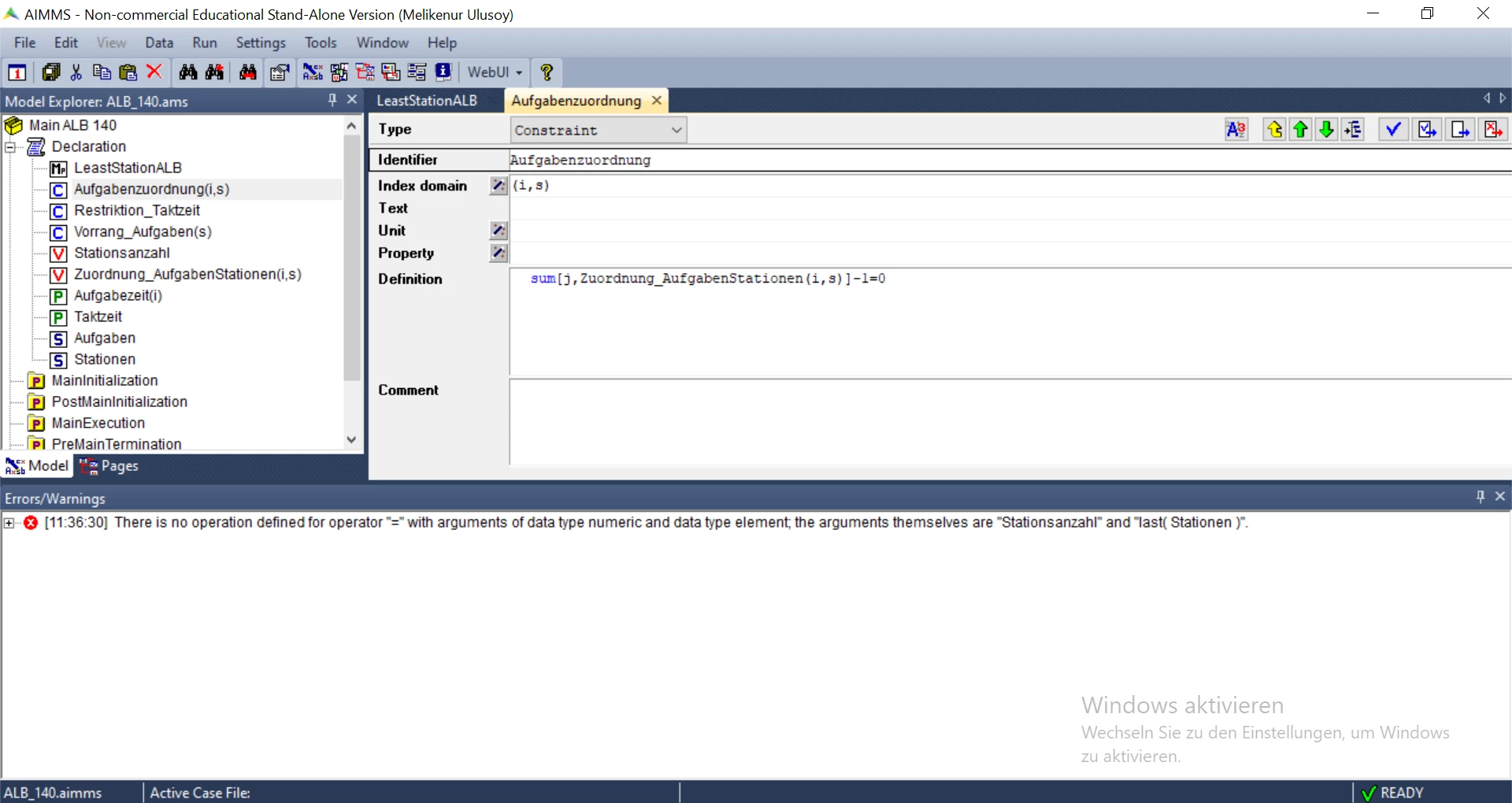Userlevel 5+5
@Melike , using Last(Stationen) as the definition for variable Stationenzahl is incorrect.

Last(Stationen) will return a set element. A variable of a math program must be a numerical quantity, not a set element.

If you want to minimize the number of stations used, I would recommend creating a binary variable bvStationUsed(s) which represents if a station is used or not. 1 = station in use, 0 = station not used.

Now, in your objective function use the definition sum[s, bvStationUsed(s)] which would give the total number of stations used.

You will also need an additional constraint restricting that a station can be assigned task only if that station is in use. So, something like

Constraint(i, s) with definition Zuordnung_AufgabenStationen(i, s) <= bvStationUsed(s)

(Assuming the i is an index in the set of tasks)
Hello Mohan,

I applied it and I have also written the execution program and added the data. hovewer after I ran the program it didnt run any iterations and I had the notification that an integer solution to the program does not exist . When does this error happen?
Thanks a lot
Userlevel 5+5
@Melike , there are a number of reasons why this could happen including some of your constraints are conflicting or the data is poor or a constraint is written wrongly.